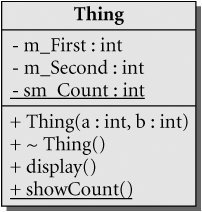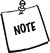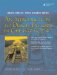# The Keyword static

The keyword static can be applied to a variable declaration to give the variable static storage class (see Section 20.3).

A local static variable is created only once, the first time its declaration statement is processed by the running program. It is destroyed when the program terminates. A static data member of a class is created once, just before the program begins execution, and is destroyed when the program terminates.

A static data member is a piece of data that is associated with the class itself rather than one that belongs to a particular object. It does not affect the sizeof() an object of the class. Each object of a class maintains its own set of non-static data members, but there is only one instance of any static data member, and it can be shared by all objects of the class.

static class members are preferable to (and can generally replace the use of) global variables because they do not pollute the global namespace.

static data members must be declared static in (and only in) the class definition.

A class member function that does not in any way access the non-static data members of the class can (and should) be declared static. In Example 2.11, the static data member is a private counter that keeps track of the number of Thing objects that exist at any given moment. The public static member function displays the current value of the static counter.

Example 2.11. src/statics/static.h

 ```[ . . . . ] class Thing { public: Thing(int a, int b); ~Thing(); void display() const ; static void showCount(); private: int m_First, m_Second; static int sm_Count; }; [ . . . . ] ```

The class UML diagram for Thing is shown in Figure 2.3. Notice that the static members are underlined in the diagram.

Figure 2.3. Thing class diagramEach static data member must be initialized (defined) outside the class definition, preferably in the class implementation file (not a header file) as shown in Example 2.12.

 The exception to this rule is a static const int, which can be initialized in the class definition.

Example 2.12. src/statics/static.cpp

 ```#include "static.h" #include int Thing::sm_Count = 0; <-- 1 Thing::Thing(int a, int b) : m_First(a), m_Second(b) { ++sm_Count; } Thing::~Thing() { --sm_Count; } void Thing::display() const { using namespace std; cout << m_First << "\$\$" << m_Second; } void Thing::showCount() { <-- 2 using namespace std; cout << "Count = " << sm_Count << endl; } ```   (1)Must initialize static member! (2)static functionNotice that the term static does not appear in the definitions of sm_Count or showCount(). This is because the keyword static would mean something quite different there: It would change the scope of the variable from global to file-scope (see Section 20.2).

Block-Scope static

statics that are defined inside a function or a block of code are initialized when they are executed for the first time.

```long nextNumber() {
int localvar(24);
static long nmber = 1000;
cout << nmber + localvar;
return ++nmber;
}
```

The first call to nextNumber() initializes localvar to 24 and nmber to 1000, displays 1124 on the screen, and returns 1001. When the function returns, localvar is destroyed but nmber is not destroyed. Each time that this function is called, localvar gets created and initialized to 24 again. The static variable nmber persists between calls and holds onto the value that it obtained in the last call. So, for example, the next time the function is called, 1025 is displayed and 1002 is returned.

static Initialization

A static object that is not defined in a block or function is initialized when its corresponding object module (see Section 7.1) is loaded for the first time. Most of the time, this is at program startup, before main() starts. The order in which modules get loaded and variables get initialized is implementation dependent.

A static object is constructed once and persists until the program terminates. A static data member is a static object that has class scope.

In Example 2.13, we make use of an internal block so that we can introduce some local objects that will be destroyed before the program ends.

Example 2.13. src/statics/static-test.cpp

 ```#include "static.h" int main() { Thing::showCount(); <-- 1 Thing t1(3,4), t2(5,6); t1.showCount(); <-- 2 { <-- 3 Thing t3(7,8), t4(9,10); Thing::showCount(); } <-- 4 Thing::showCount(); return 0; } ```   (1)No objects exist at this point. (2)direct access through object (3)An inner block of code is entered. (4)end inner block

Here is the compile and run.

```src/statics> g++ -ansi -pedantic -Wall static.cpp static-test.cpp
src/statics> ./a.out
Count = 0
Count = 2
Count = 4
Count = 2
src/statics>
```

### Copy Constructors and Assignment OperatorsAn Introduction to Design Patterns in C++ with Qt 4
ISBN: 0131879057
EAN: 2147483647
Year: 2004
Pages: 268